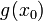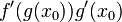# Difference between revisions of "Chain rule for higher derivatives"

Suppose$n$ is a natural number, and$f$ and$g$ are functions such that$g$ is$n$ times differentiable at$x = x_0$ and$f$ is$n$ times differentiable at$g(x_0)$. Then,$f \circ g$ is$n$ times differentiable at$x_0$. Further, the value of the$n^{th}$ derivative is given by a complicated formula involving compositions, products, derivatives, evaluations, and sums that depends on$n$.
Value of$n$ Formula for$n^{th}$ derivative of$f \circ g$ at$x_0$
1$\! f'(g(x_0))g'(x_0)$ (this is the chain rule for differentiation)
2$\! f''(g(x_0))(g'(x_0))^2 + f'(g(x_0))g''(x_0)$ (obtained by using the chain rule for differentiation twice and using the product rule for differentiation).